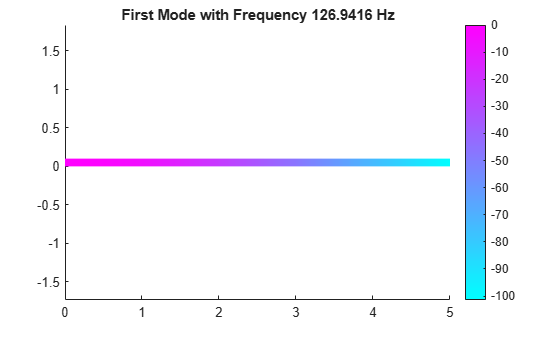# ModalStructuralResults

Structural modal analysis solution

## Description

A `ModalStructuralResults` object contains the natural frequencies and modal displacement in a form convenient for plotting and postprocessing.

Modal displacement is reported for the nodes of the triangular or tetrahedral mesh generated by `generateMesh`. The modal displacement values at the nodes appear as an `FEStruct` object in the `ModeShapes` property. The properties of this object contain the components of the displacement at the nodal locations.

You can use a `ModalStructuralResults` object to approximate solutions for transient dynamics problems. For details, see `solve`.

## Creation

Solve a modal analysis problem by using the `solve` function. This function returns a modal structural solution as a `ModalStructuralResults` object.

## Properties

expand all

Properties of an `FEStruct` object are read-only.

Natural frequencies of the structure, returned as a column vector.

Data Types: `double`

Modal displacement values at the nodes, returned as an `FEStruct` object. The properties of this object contain components of modal displacement at nodal locations.

Finite element mesh, returned as a `FEMesh` object. For details, see FEMesh Properties.

## Examples

collapse all

Find the fundamental (lowest) mode of a 2-D cantilevered beam, assuming prevalence of the plane-stress condition.

Specify the following geometric and structural properties of the beam, along with a unit plane-stress thickness.

```length = 5; height = 0.1; E = 3E7; nu = 0.3; rho = 0.3/386;```

Create a model plane-stress model, assign a geometry, and generate a mesh.

```structuralmodel = createpde('structural','modal-planestress'); gdm = [3;4;0;length;length;0;0;0;height;height]; g = decsg(gdm,'S1',('S1')'); geometryFromEdges(structuralmodel,g);```

Define a maximum element size (five elements through the beam thickness).

```hmax = height/5; msh=generateMesh(structuralmodel,'Hmax',hmax);```

Specify the structural properties and boundary constraints.

```structuralProperties(structuralmodel,'YoungsModulus',E, ... 'MassDensity',rho, ... 'PoissonsRatio',nu); structuralBC(structuralmodel,'Edge',4,'Constraint','fixed');```

Compute the analytical fundamental frequency (Hz) using the beam theory.

```I = height^3/12; analyticalOmega1 = 3.516*sqrt(E*I/(length^4*(rho*height)))/(2*pi)```
```analyticalOmega1 = 126.9498 ```

Specify a frequency range that includes an analytically computed frequency and solve the model.

`modalresults = solve(structuralmodel,'FrequencyRange',[0,1e6])`
```modalresults = ModalStructuralResults with properties: NaturalFrequencies: [32x1 double] ModeShapes: [1x1 FEStruct] Mesh: [1x1 FEMesh] ```

The solver finds natural frequencies and modal displacement values at nodal locations. To access these values, use `modalresults.NaturalFrequencies` and `modalresults.ModeShapes`.

`modalresults.NaturalFrequencies/(2*pi)`
```ans = 32×1 105 × 0.0013 0.0079 0.0222 0.0433 0.0711 0.0983 0.1055 0.1462 0.1930 0.2455 ⋮ ```
`modalresults.ModeShapes`
```ans = FEStruct with properties: ux: [6511x32 double] uy: [6511x32 double] Magnitude: [6511x32 double] ```

Plot the y-component of the solution for the fundamental frequency.

```pdeplot(structuralmodel,'XYData',modalresults.ModeShapes.uy(:,1)) title(['First Mode with Frequency ', ... num2str(modalresults.NaturalFrequencies(1)/(2*pi)),' Hz']) axis equal```